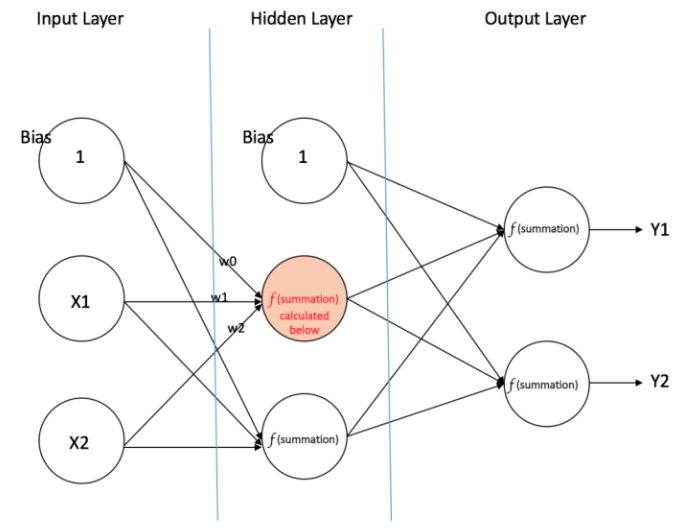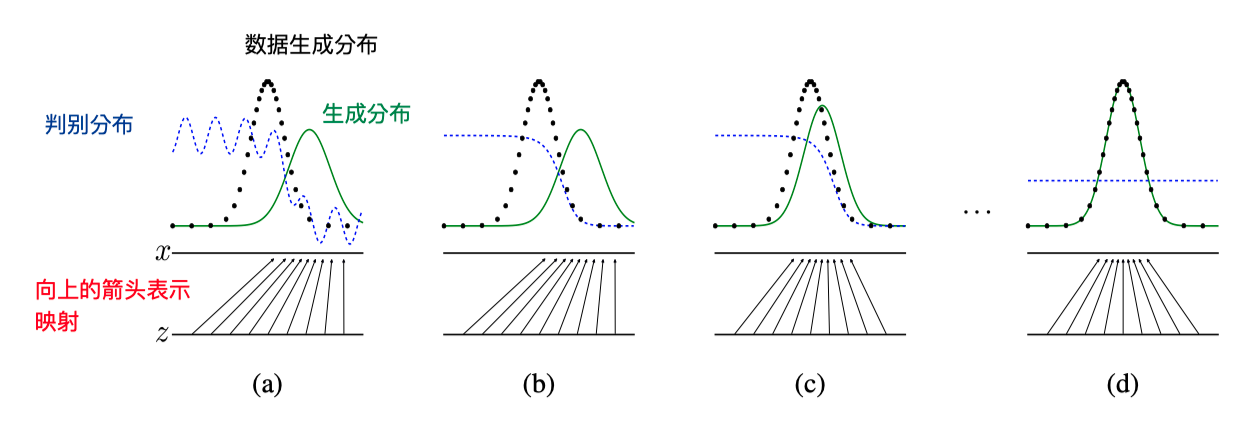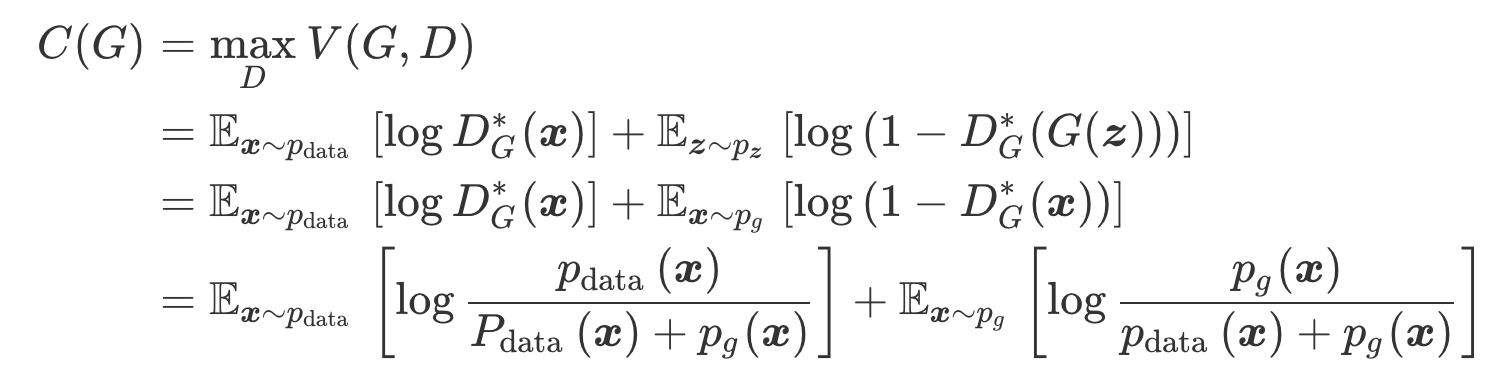## 1. Introduction

### GAN模型解决的问题

（1）最大似然估计等相关策略中难以处理的概率计算；
（2）在生成环境中难以利用分段线性单元的优势。

PS：深度生成模型是为了从原始的样本数据中模拟出数据分布，进而产生符合这一分布的新的样本。

### GAN模型的构成

GAN模型主要分为两个部分：生成模型$G$(generative model)和判别模型$D$(discriminative model)。作者将这两个部分的关系类比为假币制造商和警察。判别模型（警察）来判断一个样本究竟是来自于数据分布还是模型分布，而生产模型（假币制造商）则是为了生成假的样本来骗过判别模型（警察）。这样产生的竞争驱使两方都不断更新自己的方法，直到假样本与真样本完全无法区分。框架中的生成模型通过将随机噪声输入多层感知机进而得到假样本，而判别模型则是将样本输入多层感知机来判断样本是否为真实样本。作者指出，同时训练这两个模型的方法是使用反向传播算法(backpropagation algorithm)和丢弃算法（应该是“随机失活算法”，之前表述有所错误）(dropout algorithm)

ps：多层感知机(Multi Layer Perceptron) 为如下图所示的包括至少一个隐藏层（除去一个输入层和一个输出层以外）的多层神经元网络。• $G\left(\boldsymbol{z} ; \theta_{g}\right)$表示从噪音$\boldsymbol{z}$到数据样本空间的映射函数，其中$\theta_{g}$表示多层感知机的参数。
• $D(\boldsymbol{x};\theta_d)$表示对于输入样本$\boldsymbol{x}$输出判断为真实数据的概率（为一个标量）

### GAN的目标

$$\min_G \max_D V(D,G)=\mathbb{E}_{\mathbf{x}\sim p_{data}(x)}[\log D(x)]+\mathbb{E}_{\mathbf{z}\sim p_z}[\log (1-D(G(z)))]$$

### 图形理解• 图(a)中表示训练还未开始或刚开始一段时间时，判别模型和生成模型都还没有经过大量的训练，因此判别概率分布还有些波动，生成分布距离真实数据生成分布还有不小的距离。
• 图(b)表示经过一段时间的训练后，判别模型可以较好的判别出原始样本和生成样本，蓝色虚线的高度表示当前位置对应横坐标的样本为真实数据样本的概率，越高表示为真实样本的概率越大。
• 图(c)表示继续训练一段时间之后，原始样本和生成样本更加接近，判别模型还是有相对不错的判别效果。
• 图(d)表示经过足够的训练之后，原始样本与生成样本的概率分布特征基本一致，判别模型失去判别效果。

## 4. Theoretical Results

### 全局最优$p_g=p_{data}$